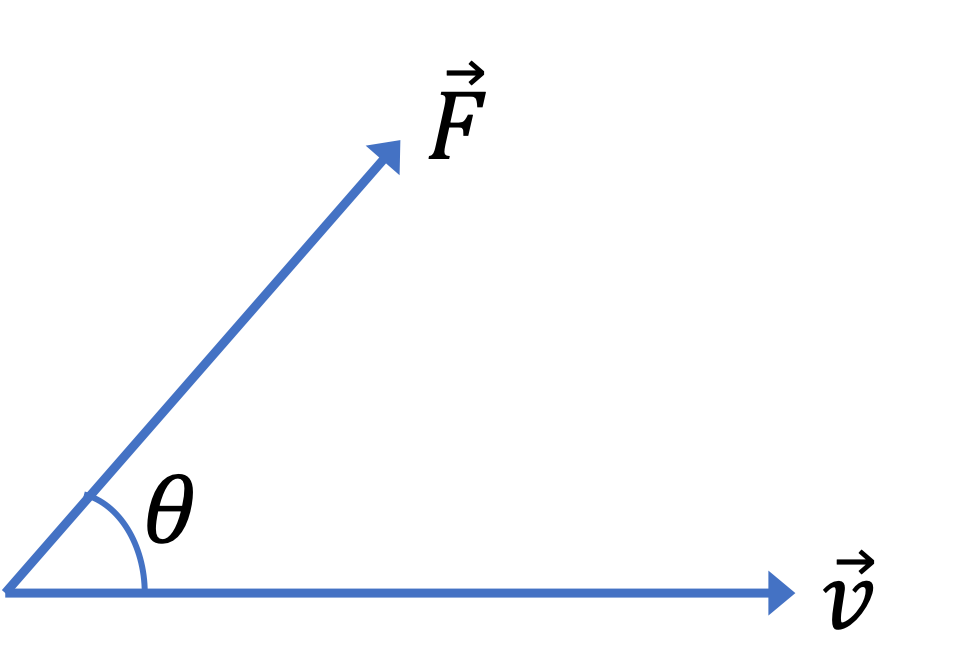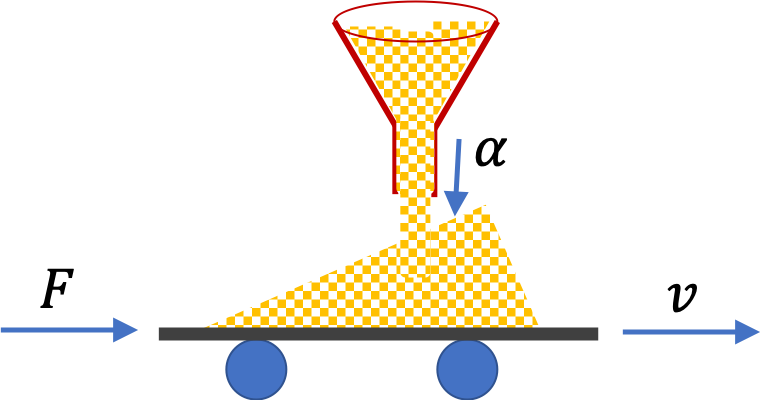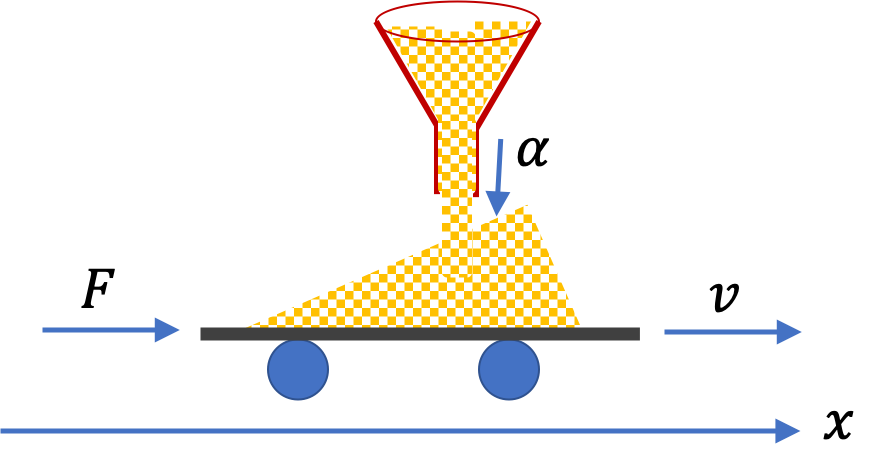## Section8.2Power of a Force

The rate at which a force does work is called power of that force. That is, if work $W_{if}$ is done over an interval of $\Delta t\text{,}$ then,

\begin{equation} \text{Power} = \text{Rate of Work} = \dfrac{W_{if}}{\Delta t}.\tag{8.2.1} \end{equation}

Since work is proportional to the magnitude of force, more work will be done if you use a larger force for the same displacement. With a larger force, you will have to do more work but you would also get the work done in less time, and, consequently, will have more power.

If force varies with time, then the rate of work will also vary with time. Therefore, we define two types of power - an instantaneous power, to be denoted by $P(t)$ and an average power during an interval $t_i$ to $t_f \text{,}$ denoted by $P_{\text{av}} \text{.}$

### Subsection8.2.1Instantaneous Power

Suppose a force $\vec F$ acts on an object which is moving with instantanous velocity $\vec v$ at instant $t \text{.}$ In duration $t$ to $t + \Delta t\text{,}$ the displacment will be $\Delta \vec r = \vec v\Delta t\text{.}$ Here we are thinking $\Delta t$ to be small, ideally infinitesimal. Therefore, work during this interval is

\begin{equation*} W = \vec F \cdot \Delta \vec r = \vec F \cdot \vec v\Delta t. \end{equation*}We omit the subscriot “$if$” from $W$ since the instants will be indistinguishable in the infinitesimal limit. Dividing $W$ by duration $\Delta t\text{,}$ and ideally taking $\Delta t\rightarrow 0$ limit in a Calculus sense, will give us the rate at which work is being done, i.e., the instantaneous power will be

\begin{equation} P(t) = \dfrac{W}{\Delta t} = \vec F \cdot \vec v.\label{eq-instantaneous-power-dot-prod-def}\tag{8.2.2} \end{equation}

As you know that the dot product can be written using magnitudes of $\vec F$ and $\vec v$ and angle $\theta$ between them or in terms of their components, giving us other formulas for the instantaneous power.

\begin{equation} P(t) = F\, v\, \cos\,\theta = F_xv_x + F_yv_y + F_zv_z.\tag{8.2.3} \end{equation}

### Subsection8.2.2Average Power

The average power is the average of this quantity over an interval.

\begin{equation} P_{\text{av}} = \text{Average of }P(t)\text{ over the interval }\Delta t.\tag{8.2.4} \end{equation}

If force and velocity are constant, then, averaging is easy to do. Say, both force and velocity are in the positive $x$ direction with fixed magnitudes. Then, the average will be same as instantaneous. Then, by performing the following series of calculations, we can obtain an alternative form of average power, which is very useful in practice.

\begin{equation*} P_{\text{av}} = F_x v_x = F_x\, \dfrac{\Delta x}{\Delta t} = \dfrac{F_x\Delta x}{\Delta t} = \dfrac{W_{if}}{\Delta t}. \end{equation*}

This demonstrates that the average power is the overall rate at which work is being perfomed by the force.

\begin{equation} P_{\text{av}} = \dfrac{W_{if}}{\Delta t}.\label{eq-average-power-work-over-time-def}\tag{8.2.5} \end{equation}

Another way to average the instantaneous power, $P(t)\text{,}$ is to plot $P(t)$ versus time. The net area under the curve between $t_i$ and $t_f$ will give the net work done during the interval. Dividing that by the interval will give the average power.

\begin{equation} P_{\text{av}} = \dfrac{ \text{Area under }P(t)\text{ versus } t }{ \Delta t }.\tag{8.2.6} \end{equation}

The SI unit of work and time gives us the unit of work as $\text{J/s}\text{,}$ which is also called Watts and abbreviated by $\text{W}\text{.}$ The unit $\text{Watts}$ is named in honor of James Watts, who made critical improvements to early steam engines.

You push a heavy machinery horizontally across the room for a total distance of $3 \text{ m}$ with a constant horizontal force of magnitude $250\text{ N}\text{.}$(a) If you completed your task in $10\text{ sec}\text{,}$ what would be your average power?

(b) You can complete the task in $5\text{ sec}\text{,}$ if you apply $1000\text{ N}\text{.}$ What would be your average power now?

Hint

(a) Use average power formula (b) calculate the new work.

(a) $75\text{ W}\text{,}$ (b) $600\text{ W} \text{.}$

Solution 1 (a)

(a) Here, we are given information to find the work done by the push.

\begin{equation*} P_{\text{av}} = \dfrac{W_{if}}{\Delta t} = \dfrac{ 250 \times 3}{10}= 75\dfrac{\text{ W}}{\text{s}} = 75\text{ W}. \end{equation*}
Solution 2 (b)

(b) Your work will be $4\times$ as much since we have $4\times$ the force in (a) for the same displacement. But you did that work $2\times$ faster. Therefore, your power will be $8\times$ as much.

\begin{equation*} P_{\text{av}} = \dfrac{ 1000 \times 3}{5} = 600\text{ W}. \end{equation*}

You drop a $10\text{-kg}$ ball from a $100\text{-m}$ tall building initially at rest.

(a) Find the instantaneous power at the following instants in time: (i) $0 \text{,}$ (ii) $2\text{ sec}\text{,}$ and (iii) $4\text{ sec}\text{.}$

(b) Find the average power between $t=0$ and $4\text{ sec}\text{.}$

Hint

Find the velocities first.

(a) (i) $0\text{,}$ (ii) $1925\text{ W}\text{,}$ (iii) $3849\text{ W}\text{,}$ (b) $1925\,\text{ W}\text{.}$

Solution 1 (a)

(a) We will use $P = \vec F\cdot \vec v\text{.}$ The force is the weight of magnitude $mg \text{,}$ down, in the same direction the velocity, therefore, the dot product will give:

\begin{equation*} P(t) = mg v. \end{equation*}

where $v$ is the speed at instant $t \text{.}$ Since the ball is falling freely at constant acceleration of $g\text{,}$ we will have

\begin{equation*} v = g t. \end{equation*}

Therefore, the instantaneous power at instant $t$ is

\begin{equation*} P(t) = mg^2 t = 10\times 9.81^2\, t = 962.36\, t. \end{equation*}

Therefore, the instantaneous power at required instants are:

\begin{equation*} \text{(i) } 0,\ \ \text{(ii) } 1925\text{ W},\ \ \text{(iii) } 3849\text{ W}. \end{equation*}
Solution 2 (b)

(b) In (a) we found that the instaneous power is

\begin{equation*} P(t) = 962.36\, t. \end{equation*}

Plot this function versus time from $t=0$ to $t=4$ and compute the area under the curve gives

\begin{equation*} P_\text{av} = \dfrac{\text{Area under } P(t) \text{ vs } t}{4\text{ sec}} = \dfrac{962.36\times \frac{4^2}{2}}{4}= 1925\,\text{ W}. \end{equation*}

Grains from a hopper falls at a rate of $10 \text{ kg/sec}$ vertically onto a conveyor belt that is moving horizontally at a constant speed $2 \text{ m/s}\text{.}$

(a) What force is needed to keep the conveyor belt moving at the constant velocity?

(b) What is the minimum power of the motor driving the conveyor belt?Hint

(a) Use $F_\text{av}\Delta t = \Delta p\text{,}$ (b) Use $P=Fv\text{.}$

(a) $20 \text{ N}\text{,}$ (b) $40 \text{ W} \text{.}$

Solution 1 (a)

The horizontal force must proivide the impulse needed to increase $x$ component of momentum of the grains. In one second, $v_x$ of $10 \text{ kg}$ of grains increases from zero to $2 \text{ m/s}\text{.}$ This gives us the momentum change.Equating $x$ impulse during one sec interval to change in $x$ momentum gives

\begin{equation*} F\times 1\text{ sec} = 10 \text{ kg}\times 2 \text{ m/s} - 0. \end{equation*}

Therefore, $F = 20\text{ N}.$

Solution 2 (b)

The power of the force will come from the dot product of the force with the velocity. This gives

\begin{equation*} P = F\,v = 40\text{ W}. \end{equation*}

### Subsection8.2.3(Calculus) Time Averaging of Instantaneous Power

The time-average of a function $f(t)$ of time $t$ over an interval from $t_i$ to $t_f$ is defined by the following integral.

\begin{equation} f_{\text{av}} = \dfrac{1}{t_f - t_i}\, \int_{t_i}^{t_f}\, f(t)\, dt.\label{eq-time-averaging-general-def}\tag{8.2.7} \end{equation}

We now show that time averaging $P(t)$ given in Eq. (8.2.2) yields the result in Eq. (8.2.5). We start with the time averaging given in (8.2.7) applied to instantaneous power $P(t) \text{.}$

\begin{align*} P_{\text{av}} \amp = \dfrac{1}{t_f - t_i}\, \int_{t_i}^{t_f}\, P(t)\, dt, \\ \amp = \dfrac{1}{t_f - t_i}\, \int_{t_i}^{t_f}\, \vec F\cdot \vec v\, dt \ \ (\text{def. of }P(t)),\\ \amp = \dfrac{1}{t_f - t_i}\, \int_{t_i}^{t_f}\, \vec F\cdot \dfrac{d\vec r}{dt}\, dt \ \ (\text{def. of }\vec v),\\ \amp = \dfrac{1}{t_f - t_i}\, \int_{i}^{f}\, \vec F\cdot d\vec r \ \ (\text{cancel }dt), \\ \amp = \dfrac{1}{t_f - t_i}\, W_{if}, \ \ (\text{def. of }W_{if}), \end{align*}

which was to be proved.

You drop a $10\text{-kg}$ ball from a $100\text{-m}$ tall building initially at rest. Find the average power during the following intervals: (i) $t_i = 0$ to $t_f = 2\text{ sec}\text{,}$ and (ii) $t_i = 2\text{ sec}$ to $t_f=4\text{ sec}\text{.}$

Hint

First derive the instantaneous power function of time. then use the time averaging process to obtain the average power.

(i) $962\text{ W}\text{,}$ (ii) $2887\text{ W}$

Solution

Above, in Checkpoint 8.2.2, we found that the instantaneous power for a ball dropped from rest is

\begin{equation*} P(t) = mg^2 t. \end{equation*}

Time average of this function will be

\begin{align*} P_{\text{av}}\amp = \dfrac{1}{t_f-t_i}\int_{t_i}^{t_f}\, P(t)\, dt = \dfrac{mg^2}{2(t_f-t_i)} \left( t_f^2 - t_i^2\right),\\ \amp = \dfrac{mg^2 (t_f+t_i)}{2} = 481.18 \left( t_f + t_i\right). \end{align*}

(i) We now use the numerical values of the times we get

\begin{equation*} P_{\text{av}} = 481.18 \times (2+0) = 962\text{ W}. \end{equation*}

(ii) We now use the numerical values of the times we get

\begin{equation*} P_{\text{av}} = 481.18 (4+2) = 2887\text{ W}. \end{equation*}